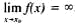# Infinitely Large Quantity

The following article is from The Great Soviet Encyclopedia (1979). It might be outdated or ideologically biased.

## Infinitely Large Quantity

in mathematics, a variable quantity that during a given process of variation becomes and remains greater in absolute value than any preas-signed number. The study of infinitely large quantities can be reduced to the study of infinitesimals, because if y is an infinitely large quantity, then its reciprocal z = 1/y is an infinitesimal. The fact that the variable y is an infinitely large quantity is written in the form limy = ∞. Here, the symbol ∞ (“infinity”) is only a conventional symbol indicating thaty is an infinitely large quantity. Another point of view is also possible according to which ∞ is an improper element added to the set of real numbers. Applicable to the function of an argument x, there is the following definition of the infinitely large quantity: the function f(x), defined in the neighborhood of the point x0, is said to be infinitely large for x approaching x0, if for any number N > 0 there exists a number δ > 0 such that for all x = x0, ǀx - x0ǀ < δ, then the inequality ǀf(x)ǀ > N is satisfied. This property is written in the form.

S. B. STECHKIN